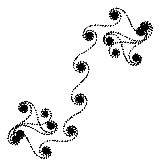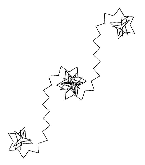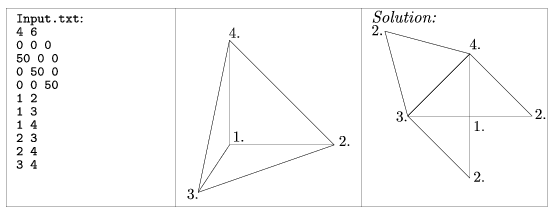Mathematical and Physical Journal
for High Schools
Issued by the MATFUND Foundation
 Already signed up? New to KöMaL?

# KöMaL Problems in Informatics, December 2006

Show/hide problems of signs:## Problems with sign 'I'

Deadline expired on January 15, 2007.

I. 145. Prepare a database for a certain informatics company and answer the questions below. The first table of the database ITEMS contains some hardware and software items: ID number, name and unit selling price of the item. The second table of the database IMPORT gives information on the purchase and suppliers: ID number of an item, unit price, date of purchase, number of items purchased, and ID number of supplier. The third table SUPPL contains further information on the suppliers: ID number, name, location (city) of the supplier, and name of the contact person. The questions to be answered or completed are the following:

a) make the table ITEMS;

b) make the table IMPORT;

c) make the table SUPPL;

d) list the names and locations of suppliers of items containing the word ''modem'';

e) list the names of suppliers shipping items of unit price below 1500 HUF;

f) list the names of items that can be bought from at least three suppliers;

g) list the ID number, name and total number of each item;

h) for each supplier, give the number of items with total value that can be bought from them. This list should contain the number of items ordered in descending order;

i) list those contact persons with company names that have not shipped anything for more than a year;

j) make a list of each item, containing the name, ID number, and cheapest unit price with the name of the corresponding supplier. The list should contain the names of items in ascending order.

A text file I145.txt should be submitted, with each line containing the appropriate SQL commands solving the questions above.

(10 pont)

solution (in Hungarian), statistics

I. 146. Make a program displaying a range of colours on a web page. The input of your program consists of the range (starting colour number, final colour number, both being hexadecimal HTML codes, e.g. A160BB) and the increment between adjacent colours displayed (a decimal number, e.g. 120). The colours should be displayed in increasing order, regardless of the order of the input parameters. The output i146.html should display a table with 8 columns, each cell containing the colour code in black letters with the actual background colour.

The source code of the program (i146.pas, i146.cpp, ...) generating the HTML page is to be submitted.

(10 pont)

solution (in Hungarian), statistics

I. 147. The following algorithm generates some spiral-like figures. Consider a horizontal line segment, then, in every step, the starting point of the next, nth line segment and the endpoint of the previous one is joined in such a way that these segments encompass an angle of bn, where numbers bn are members of an arithmetic progression bn-bn-1=d with constant d. In the construction each segment has the same length.

Prepare your program to display these spirals. The input consists of the number d (real number) and the common length L (positive real number) of the segments. A message should notify the user when all possible line segments have been drawn (that is, when repetition occurs). See the example.d=24,01   L=1,5d=177   L=10

The source code of the program (i147.pas, i147.cpp, ...) should be submitted.

(10 pont)

solution (in Hungarian), statistics## Problems with sign 'S'

Deadline expired on January 15, 2007.

S. 22. In this exercise your program should draw the planar net of a given convex polyhedron.

A convex polyhedron is a 3 dimensional solid bounded by planes and its vertices and edges will be given. The net of the polyhedron should be cut at appropriate points and drawn in the plane without distortion. It is required that polygons in the planar net and corresponding faces of the polyhedron be congruent. The planar net should not overlap itself and should be connected.

The name of the input file is given as a command line argument as s22.exe Input.txt.

The first line of the input file contains two integers 3<n<30 and 6<k<300. Then 3 integers separated by spaces stand in each of the following n lines, representing the (x,y,z) coordinates of a vertex. The next k lines contain 2 integers each, representing the numbers of vertices on the same edge of the polyhedron. The smallest vertex number is 1, referring to the vertex in the second line of the input file.

Example:The source code of the program (s22.pas, s22.cpp, ...) with some remarks and a short documentation (s22.txt, s22.pdf, ...) should be submitted.

(10 pont)

solution (in Hungarian), statistics

\$Var(Body)